## Math 480 Course Notes -- May 22, 1996

### Crash Course on Probability

The basic concept in probability theory is that of a random variable. A random variable is a function of the basic outcomes in a probability space. To define a probability space (a la Kolmorogov) one needs three ingredients:

1. A set S of "outcomes" for some experiment. This is the set of all "basic" things that can happen. This set can be a discrete set (such as the set of 5-card poker hands, or the possible outcomes of rolling two dice) or it can be a continuous set (such as an interval of the real number line for measuring temperature, etc).
2. A sigma-algebra of subsets of S. This means a set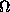of subsets of S (soitself is a subset of the power set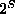of all subsets of S) that contains the empty set, contains S itself, and is closed under finite intersections and countable unions. When the basic set S is finite (or countably infinite), thenis taken to be all subsets of S. When S is a continuous subset of the real line, this is not possible, and one usually restricts attention to the set of subsets that can be obtained by starting with all open intervals and taking intersections and unions (countable) of them -- the so-called Borel sets [or more generally, Lebesgue measurable subsets of S].is the collection of sets to which we will assign probabilities.
3. A function p fromto the real numbers that assigns probabilities to events. The function p must have the properties that:
1. p(S)=1, p()=0.
2.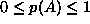for every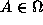3. If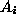, i=1,2,3,.... is a countable (finite or infinite) collection of disjoint sets (i.e.,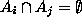for all i different from j), then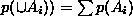.

These axioms imply that ifis the complement of A, then p()=1-p(A), and the principle of inclusion and exclusion: p(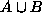)=p(A)+p(B)-p(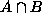), even if A and B are not disjoint.

### Examples:

Discrete Random Variables -- take on only isolated (discrete) values, such as when you are counting something. Usually the values are (subset of the) integers, and we can assign a probability to any subset of the sample space, as soon as we know the probability of any set containing one element, i.e., p({k}) for all k. Usually, we are sloppy about the notation and express this as a function p(k) --- and we set p(k)=0 for all numbers not in the sample space. We repeat, for discrete random variables, the value p(k) represents the probability that the event {k} occurs. So any function from the integers to the (real) interval [0,1] that has the property that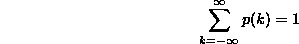defines a discrete probability distribution.

#### Finite Discrete Random Variables:

• 1. Uniform distribution -- This is often encountered, e.g., coin flips, rolls of a single die, other games of chance: S is the set of whole numbers from a to b (this is a set with b-a+1 elements!),is the set of all subsets of S, and p is defined by giving its values on all sets consisting of one element each (since then the rule for disjoint unions takes over to calculate the probability on other sets). "Uniform" means that the same value is assigned to each one-element set. Since p(S)=1, the value that must be assigned to each one element set is 1/(b-a+1).

For example, the possible outcomes of rolling one die are {1}, {2}, {3}, {4}, {5} and {6}. Each of these outcomes has the same probability, namely 1/6. We can express this by making a table, or specifying a function f(k)=1/6 for all k = 1,2,3,4,5,6 and f(k)=0 otherwise. Using the disjoint union rule, we find for example that p({1,2,5})=1/2, p({2,3})=1/3, etc..

• 2. Binomial distribution -- flip n fair coins, how many come up heads? i.e., what is the probability that k of them come up heads? Or do a sample in a population that favors the Democrat over the Republican 60 percent to 40 percent. What is the probability that in a sample of size 100, more than 45 will favor the Republican?

The sample space S is 0,1,2,....n since these are the possible outcomes (number of heads, number of people favoring the Republican [n=100 in this case]). As before, the sigma algebrais the set of all subsets of S. The function p is more interesting this time: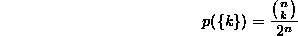where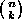is the binomial coefficient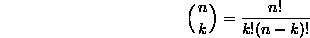which equals the number of subsets of an n-element set that have exactly k elements.

#### Infinite Discrete Random Variables:

• 3. Poisson distribution (with parameter) -- this arises as the number of (random) events of some kind (such as people lining up at a bank, or Geiger-counter clicks, or telephone calls arriving) per unit time. The sample space S is the set of all nonnegative integers S=0,1,2,3,...., and againis the set of all subsets of S. The probability function onis derived from: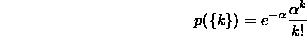Note that this is an honest probability function, since we will have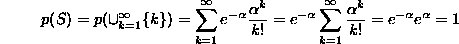Continuous Random Variables: can take on only any real values, such as when you are measuring something. Usually the values are (subset of the) reals, and for technical reasons, we can only assign a probability to certain subsets of the sample space (but there are a lot of them). These subsets, either the collection of Borel sets (sets that can be obtained by taking contable unions and intersections of intervals) or Lebesgue-measurable sets (Borels plus a few other exotic sets) comprise the set. As soon as we know the probability of any interval, i.e., p([a,b]) for all a and b, we can calculate the probabililty of any Borel set. In fact, it is enough to know the probabilities of "very small" intervals of the form [x,x+dx]. In other words, we can calculate continuous probabilities as integrals of "probability density functions" (pdf's).

A pdf is a function p(x) that takes on only positive values (they don't have to be between 0 and 1 though), and whose integral over the whole sample space (we can use the whole real line if we assign the value p(x)=0 for points x outside the sample space) is equal to 1. In this case, we have (for small dx) that p(x)dx represents (approximately) the probability of the set (interval) [x,x+dx] (with error that goes to zero faster than dx does). More generally, we have the probability of the set (interval) [a,b] is: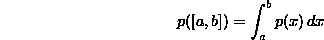So any positive function on the real numbers that has the property that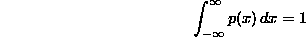defines a continuous probability distribution.

• 1. Uniform distribution. As with the discrete uniform distribution, the variable takes on values in some interval [a,b] and all variables are equally likely. In other words, all small intervals [x,x+dx] are equally likely as long as dx is fixed and only x varies. That means that p(x) should be a constant for x between a and b, and zero outside the interval [a,b]. What constant? Well, to have the integral of p(x) come out to be 1, we need the constant to be 1/(b-a). It is easy to calculate that if a< r< s < b, then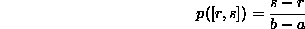• 3. (this is numbered 3 because it corresponds to the Poisson distribution). Exponential distribution (with parameter). This arises when measuring waiting times until an event, or time-to-failure in reliability studies. For this distribution, the sample space is the positive part of the real line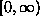(or we can just let p(x)=0 for x<0). The probability function is given by. It is easy to check that the integral of p(x) from 0 to infinity is equal to 1, so p(x) defines a bona fide probability density function. Here are graphs of exponential distribution functions with parameters 1, 1.5 and 2: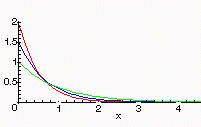• 2. (corresponds somewhat to the binomial distribution). The Normal Distribution (or Gaussian distribution). This is the most important probability distribution, because the distribution of the average of the results of repeated experiments always approaches a normal distribution (this is the "law of large numbers"). The sample space for the normal distribution is always the entire real line. But to begin, we need to calculate an integral: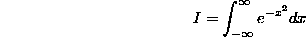We will use a trick that goes back (at least) to Liouville: First, note that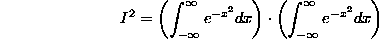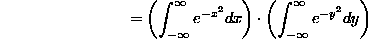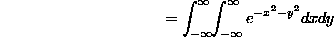because we can certainly change the name of the variable in the second integral, and then we can convert the product of single integrals into a double integral. Now (the critical step), we'll evaluate the integral in polar coordinates (!!) -- note that over the whole plane, r goes from 0 to infinity asgoes from 0 to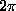, and dxdy becomes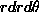: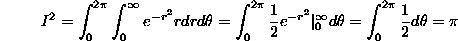Therefore, I=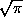. We need to arrange things so that the integral is 1, and for reasons that will become apparent later, we arrange this as follows: define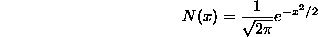Then N(x) defines a probability distribution, called the standard normal distribution. Here is a graph of N(x):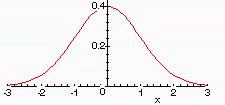More generally, we define the normal distribution with parameters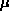andto be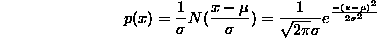### Expectation of a Random Variable

The expectation of a random variable is essentially the average value it is expected to take on. Therefore, it is calculated as the weighted average of the possible outcomes of the random variable, where the weights are just the probabilities of the outcomes. As a trivial example, consider the (discrete) random variable X (outcomes of some probabilistic experiment) whose sample space is the set {1,2,3} with probability function given by p(1)=0.3, p(2)=0.1 and p(3)=0.6. If we repeated this experiment 100 times, we would expect to get about 30 occurrences of X=1, 10 of X=2 and 60 of X=3. The average X would then be ((30)(1)+(10)(2)+(60)(3))/100 = 2.3. In other words, (1)(0.3)+(2)(0.1)+(3)(0.6). This reasoning leads to the defining formula: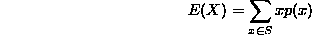for any discrete random variable. The notation E(X) for the expectation of X is standard, also in use is the notation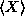.

For continuous random variables, the situation is similar, except the sum is replaced by an integral (think of summing up the average values of x by dividing the sample space into small intervals [x,x+dx] and calculating the probability p(x)dx that X falls into the interval. By reasoning similar to the previous paragraph, the expectation should be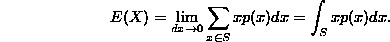This is the formula for the expectation of a continuous random variable.

### Examples:

• 1. Uniform discrete: We'll need to use the formula for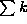that you learned in freshman calculus when you evaluated Riemann sums: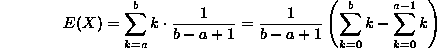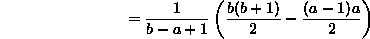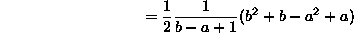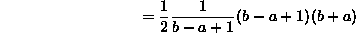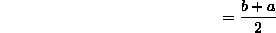which is what we should have expected from the uniform distribution.

• 2. Uniform continuous: (We expect to get (b+a)/2 again, right?). This is easier: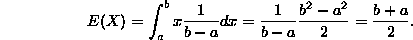• 3. Poisson distribution with parameter: Before we do this, recall the Taylor series formula for the exponential function: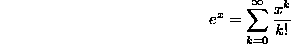Note that we can take the derivative of both sides to get the formula: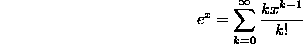If we multiply both sides of this formula by x we get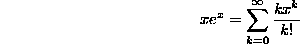We will use this formula with x replaced by.

If X is a discrete random variable with a Poisson distribution, then its expectation is: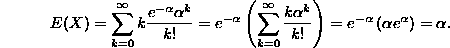• 4. Exponential distribution with parameter. This is a little like the Poisson calculation (with improper integrals instead of series), and we will have to integrate by parts (we'll use u=x so du=dx, and dv=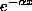dx so that v will be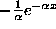):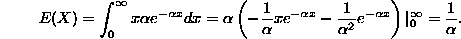Note the difference between the expectations of the Poisson and exponential distributions!!

By the symmetry of the respective distributions around their "centers", it is pretty easy to conclude that the expectation of the binomial distribution (with parameter n) is n/2, and the expectation of the normal distribution (with parametersand) is.

### Homework problems:

1. Make your own example of a probability space that is finite and discrete. Calculate the expectation of the underlying random variable X.

2. Make your own example of a probability space that is infinite and discrete. Calculate the expectation of the underlying random variable X.

3. Make your own example of a continuous random variable. Calculate its expectation.

4. Prove that the normal distribution function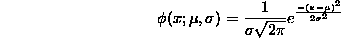is really a probability density function on the real line (i.e., that it is positive and that its integral from -infinity to infinity is 1). Calculate the expectation of this random variable.

5. What is the relationship among the following partial derivatives?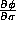,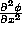, and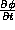, where(for the last one, rewrite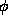as a function of x,and t).

6. Consider the experiment of picking a point at random from a uniform distribution on the disk of radius R centered at the origin in the plane ("uniform" here means if two regions of the disk have the same area, then the random point is equally likely to be in either one). Calculate the probability density function and the expectation of the random variable D, defined to be the distance of the random point from the origin.

Dennis DeTurck
Mon May 27 13:57:15 EDT 1996